Note: before reading this, you should know what a monad is. Read this post if you don't!

Here's a function `half`:And we can apply it a couple of times:

``````half . half \$ 8
=> 2
``````

Everything works as expected. Now you decide that you want to log what happens in this function: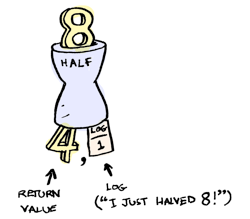``````half x = (x `div` 2, "I just halved " ++ (show x) ++ "!")
``````

Okay, fine. Now what if you want to apply half a couple of times?

``````half . half \$ 8
``````

Here's what we want to have happen: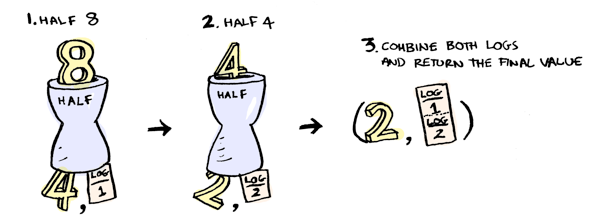Spoilers: it doesn't happen automatically. You have to do it yourself:

``````finalValue = (val2, log1 ++ log2)
where (val1, log1) = half 8
(val2, log2) = half val1
``````

Yuck! That's nowhere as nice as:

``````half . half \$ 8
``````

And what if you have more functions that log things? There's a pattern here: for each function that returns a log along with a value, we want to combine those logs. This is a side-effect, and monads are great at side effects!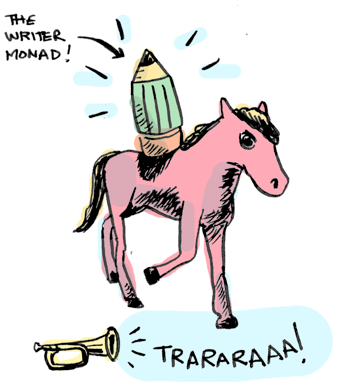The Writer monad is cool. "Hey dude, I'll handle the logging," says Writer. "Go back to your clean code and crank up some Zeppelin!"

Every writer has a log and a return value:``````data Writer w a = Writer { runWriter :: (a, w) }
``````

Writer lets us write code like this:

``````half 8 >>= half
``````

Or you can use the `<=<` function, which does function composition with monads, to get:

``````half <=< half \$ 8
``````

which is pretty darn close to `half . half \$ 8`. Cool!

You use `tell` to write something to the log. And `return` puts a value in a Writer. Here's our new `half` function:

``````half :: Int -> Writer String Int
half x = do
tell ("I just halved " ++ (show x) ++ "!")
return (x `div` 2)
``````

It returns a `Writer`:And we can use `runWriter` to extract the values from the `Writer`:``````runWriter \$ half 8
=> (4, "I just halved 8!")
``````

But the cool part is, now we can chain calls to `half` with `>>=`:

``````runWriter \$ half 8 >>= half
=> (2, "I just halved 8!I just halved 4!")
``````

Here's what's happening:`>>=` magically knows how to combine two writers, so we don't have to write any of that tedious code ourselves! Here's the full definition of `>>=`: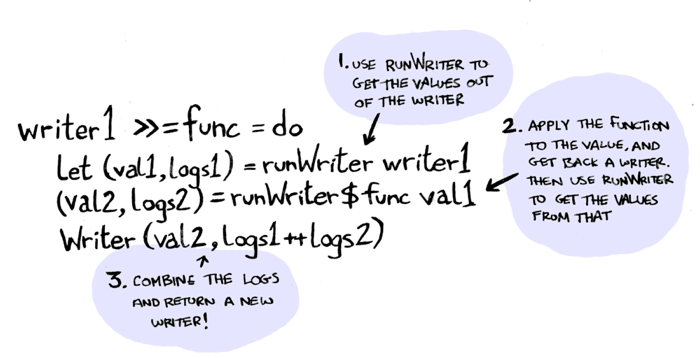Which is the same boilerplate code we had written before. Except now, `>>=` takes care of it for us. Cool! We also used `return`, which takes a value and puts it in a monad: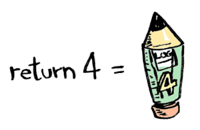``````return val = Writer (val, "")
``````

(Note: these definitions are almost right. The real `Writer` monad allows us to use any `Monoid` as the log, not just strings. I have simplified it here a bit).

Suppose you want to pass some config around to a lot of functions. Use the `Reader` monad:The reader monad lets you pass a value to all your functions behind the scenes. For example:

``````greeter :: Reader String String
greeter = do
return ("hello, " ++ name ++ "!")
``````

`greeter` returns a Reader monad:``````data Reader r a = Reader {  runReader :: r -> a }
``````

Reader was always the renegade. The wild card. Reader is different because it's only field is a function, and this is confusing to look at. But we both understand that you can use `runReader` to get that function: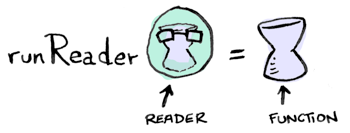And then you give this function some state, and it's used in `greeter`: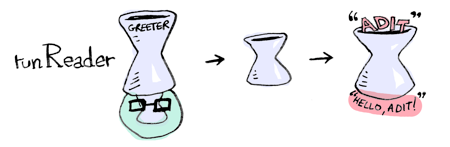``````runReader greeter \$ "adit"
``````

So when you use `>>=`, you should get a `Reader` back. When you pass in a state to that reader, it should be passed through to every function in that monad.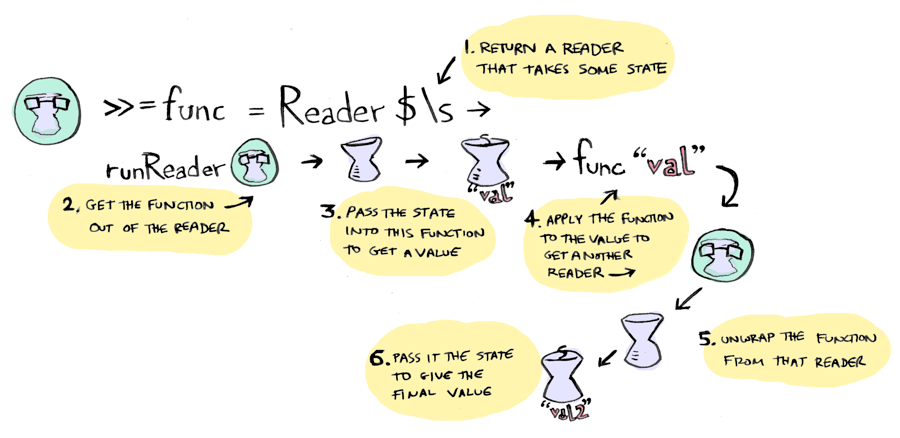``````m >>= k  = Reader \$ \r -> runReader (k (runReader m r)) r
``````

Reader always was a little complex. The complex ones are the best.

`return` puts a value in a `Reader`:``````return a = Reader \$ \_ -> a
``````

And finally, `ask` gives you back the state that was passed in:

``````ask = Reader \$ \x -> x
``````

Want to spend some more time with `Reader`? Turn up the punk rock and see this longer example.Here's how `State` is defined:

``````State s a = State { runState :: s -> (a, s) }
``````You can get the state with `get`, and change it with `put`. Here's an example:

``````greeter :: State String String
greeter = do
name <- get
put "tintin"
return ("hello, " ++ name ++ "!")

``````

Nice! Reader was all like "you won't change me", but State is committed to this relationship and willing to change.

The definitions for the `State` monad look pretty similar to the definitions for the `Reader` monad:

`return`:``````return a = State \$ \s -> (a, s)
``````

`>>=`: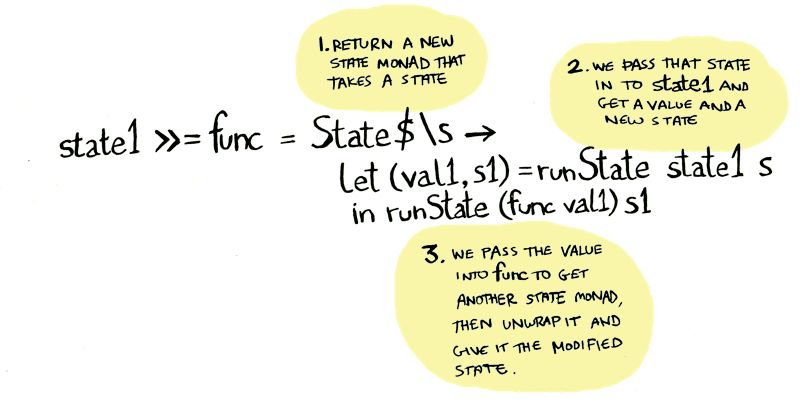``````m >>= k = State \$ \s -> let (a, s') = runState m s
in runState (k a) s'
``````

## Conclusion